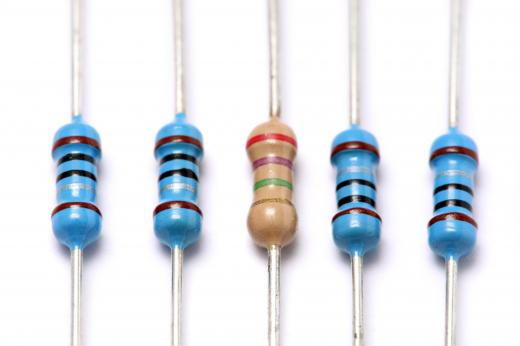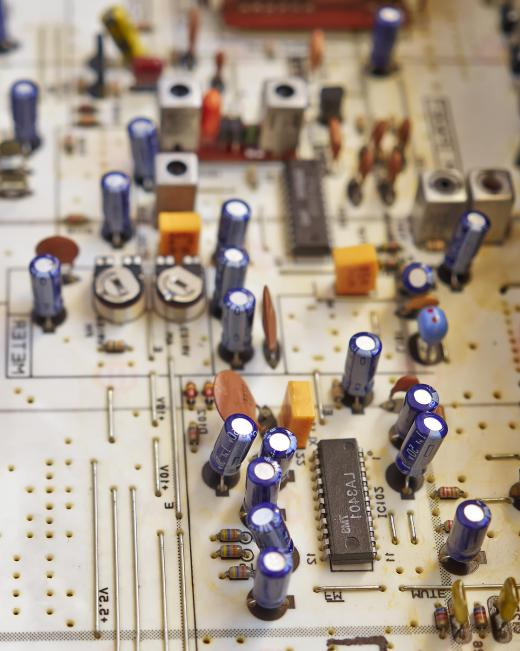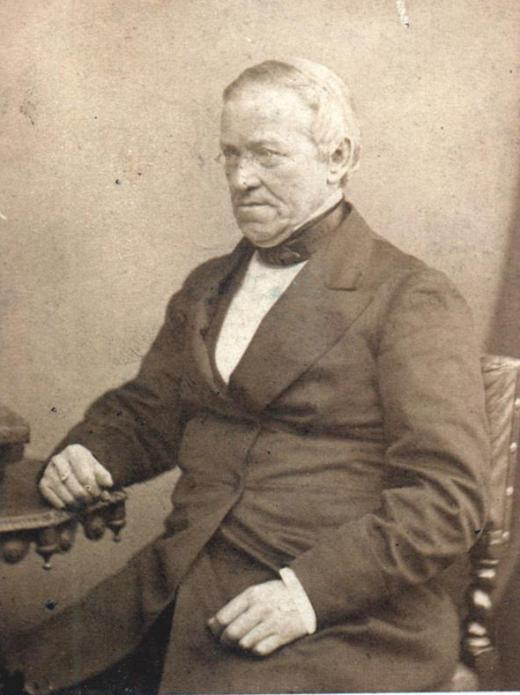# What is an Impedance Bridge?

Ron Davis
Ron DavisCarbon resistors can be used to form a resistor bridge.

A modern impedance bridge is a device used to measure impedance, capacitance, or inductance. The grandfather of the generalized impedance bridge is the Wheatstone bridge, an electrical circuit devised by Samuel Christie in 1833 and popularized some ten years later by Sir Charles Wheatstone. Wheatstone bridges, with their characteristic diamond circuit diagrams, use two fixed impedance resistors and a variable resistor to measure the unknown impedance of a fourth resistor.

The Wheatstone bridge is a direct current (DC) device. A modern device can be switched from DC to alternating current (AC) and back again. Both the Wheatstone bridge and its modern descendant, the generalized impedance bridge, work on the same principle, which is the balancing of an electrical circuit. The basic idea is that if a circuit contains two resistors of known value, a variable resistor and a resistor of unknown value can be used to determine the value of the unknown resistor, and a measuring device known as a potentiometer is used to ascertain when the variable resistor has reached the balance point for the circuit. In equation form, R1/R2 = R(u)/ Rvar where R1 and R2 are resistors of known value, R(u) is the resistor with unknown impedance and Rvar is the variable resistor with a display to show its impedance at any point in time.A resistor is an electronic component that can lower a circuit’s voltage and its flow of electrical current.

Modern impedance bridges have several circuits. One circuit is likely to be the original test circuit, with a read-out in Ohms, also known as an Ohm meter. Other circuits may include different combinations of resistors, capacitors, inductance coils, and perhaps a signal generator and a power source. With these circuits, the modern impedance bridge can test many electrical devices and circuits, from resistors and capacitors up through the tuning of an antenna. AC circuits are a little trickier than DC circuits because the circuit won’t balance until the phase of the AC is the same on both sides of the bridge.Sir Charles Wheatstone popularized the wheatstone bridge, which was a precursor to the impedance bridge.

A potential problem for the sensitive AC bridge circuits is that capacitors tend to ‘leak’ current. Stray current from capacitor leakage will generate incorrect readings at the detector or meter. A solution to the problem is to add what is known as a Wagner earth circuit. The Wagner earth circuit is a grounded voltage divider designed to have the voltage ratio and phase shift as each side of the bridge. Often there is a two position switch to allow the user to confirm the circuit has been set properly, and when the potentiometer registers zero in both switch positions, an error free reading is guaranteed.